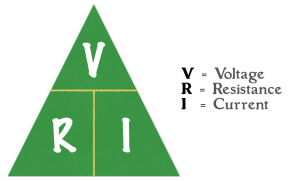Getting started with electronics always involves a discussion of Ohm’s Law. What is this mysterious sounding law and how can you use it when building electronic projects?  One the main uses for Ohm’s Law in your projects is to calculate the resistor value needed for a LED.  This article takes a look at what Ohm’s Law is and how to use it with LEDs.

# Overview

Ohm’s Law is really a mathematical rule based on two points.  In electronics those two points have a voltage across them (aka potential difference).  The amount of current that flows from one point to the other, is going to depend on the resistance between those points.   So Ohm’s law says that the Resistance of a device is equal to the Voltage Across it divided by the Current that Flows through it.  The formula looks like this:

$latex Resistance=\frac{Voltage}{Current}&s=3$

Using a little bit of algebra, you can determine the voltage drop across a device if you know the resistance and current.  Or, you can determine how much current will flow if you know the voltage and resistance.  What’s an easy way to remember this?  Well, use the  “Ohm’s Law Triangle.”

## Ohm’s Law TriangleUsing this triangle it is possible to create each of the following equations:

$latex Resistance=\frac{Voltage}{Current}&s=3$

$latex Current=\frac{Voltage}{Resistance}&s=3$

$latex Voltage=Resistance\times Current&s=3$

# Using Ohm’s Law with Current Limiting Resistors

As discussed in my introduction to LEDs (found here), you should always limit the current when using LEDs.  The simplest method, but least efficient, is to use a resistor in series with the LED.  Let’s use the example of connecting a LED to a pin on the Arduino.  When you are trying to find a current limiting resistor for a LED, you know two things about the “circuit”:  the voltage applied to the resistance and how much current is flowing through it.

## Arduino LED ExampleWhen selecting a resistor for a LED, you generally know two things:  Voltage and Current.  Here’s how you determine each.

### Voltage

The main voltage of an Arduino Uno is 5V, which means the pins will output approximately 5V when configured as an OUTPUT and set to HIGH.  So we know the voltage.   Now, what we need to calculate is the voltage drop of the current limiting resistor.  Since the resistor and LED are in series, we subtract the Forward Voltage of the LED from the Supply Voltage.

Tip:  The forward voltage of a LED will depend on its color.

We know the pin is 5V and in this example our LED has a forward voltage of 2V.

This means the Voltage across the resistor is 3V.

### Current

Typically when people use LEDs in their projects, they’ll use the maximum forward current allowed by a LED.  It isn’t necessary to use this value.  Backing away from the maximum will reduce the brightness of the LED and extend its life.  Since most people use the max, and typical LEDs are around 20mA, we’ll use 20mA for the current.

This means the Current flowing through the resistor is 20mA.

## Calculating Resistance

So our resistor will have 3V dropped across and 20mA through it.  Using the Ohm’s Law Triangle, here’s the information we know.

This shows that we are going to divide voltage by current, using this formula:

$latex Resistance=\frac{Voltage}{Current}&s=3$

Simply replace the words with numbers to get

$latex Resistance=\frac{3V}{0.02A}=150\Omega&s=3$

Which gives us a resistance of 150Ω!

# Conclusion

Calculating the resistance value of a current limiting resistor is just one use of Ohm’s Law.  You could also use the reverse to determine how current is flowing through an LED.  Now, keep in mind, that Ohm’s Law always applies, but not in ways you might expect.  The properties of Ohm’s Law is straight foward for a device like a Resistor.  However, active devices like Microprocessors aren’t simplistic enough to just use Ohm’s Law to describe their behavior.Fan of making things beep, blink and fly. Created AddOhms. Stream on Twitch. Video Host on element14 Presents and writing for Hackster.IO. Call sign KN6FGY.

This site uses Akismet to reduce spam. Learn how your comment data is processed.